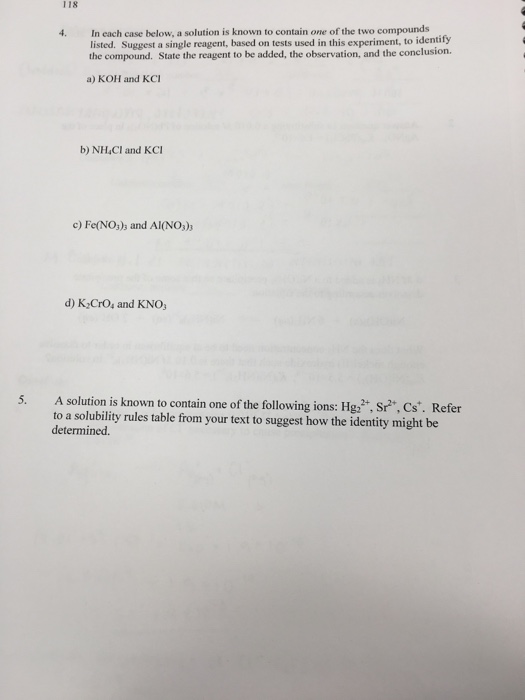# Question & Answer: In each case below, a solution is known to contain one of the two compounds listed. Suggest a…..In each case below, a solution is known to contain one of the two compounds listed. Suggest a single reagent, based on tests used in this experiment, to identify the compound. State the reagent to be added, the observation, and the conclusion. a) KOH and KCI b) NH_4Cl and KCl c) Fe(NO_3)_3 and Al(NO_3)_3 d) K_2CrO_4 and KNO_3 A solution is known to contain one of the following ions: Hg_2^2+, Sr^2+, Cs^+. Refer to a solubility rules table from your text to suggest how the identity might be determined.

Question 4.

a)

Don't use plagiarized sources. Get Your Custom Essay on
Question & Answer: In each case below, a solution is known to contain one of the two compounds listed. Suggest a…..
GET AN ESSAY WRITTEN FOR YOU FROM AS LOW AS \$13/PAGE

KOH = strong base, will have a high pH, if we add HCl, expect neutralization, heat must be released, i.e. T will increase

KCl = this is ionic salt K+ and Cl- will be in solution, Add Hg2+ ions in order to precipitate HgCl2. If solid forms, then this is ionic.

Also, flame test shoudl show K+ ion color in flames as purple

b)

NH4Cl = this is slightly acidic, should react with any base to form NH3(g) + OH- + H+ = H2O

KCl = use flame test, if violet flame is seen, this contains K+ ions

c)

Fe(NO3)3

Fe3+ ions can be detected by:

Fe(NO3)3 + 6 KSCN = K3[Fe(SCN)6] + 3 KNO3

the solution complex will form a dark -red color

For Al(NO3)

Al3+ is present, it must be in basic media so:

The aluminum ion gets involved the adsorption of the red dye aluminon (which is actually the species – aurin tricarboxylic acid).

The Al(OH)3 will be seen as a precipitate with this dye.

d)

K2CrO4 = 2K+ + CrO4-2

Add Ag+ ions, they will preicpitate with

2Ag+ + CrO4-2 = Ag2CrO4(s)

if no precipitate forms, this should be the KNO3 solution.

Use flame test to identify its color (violet) and presence